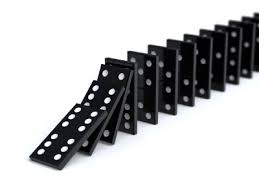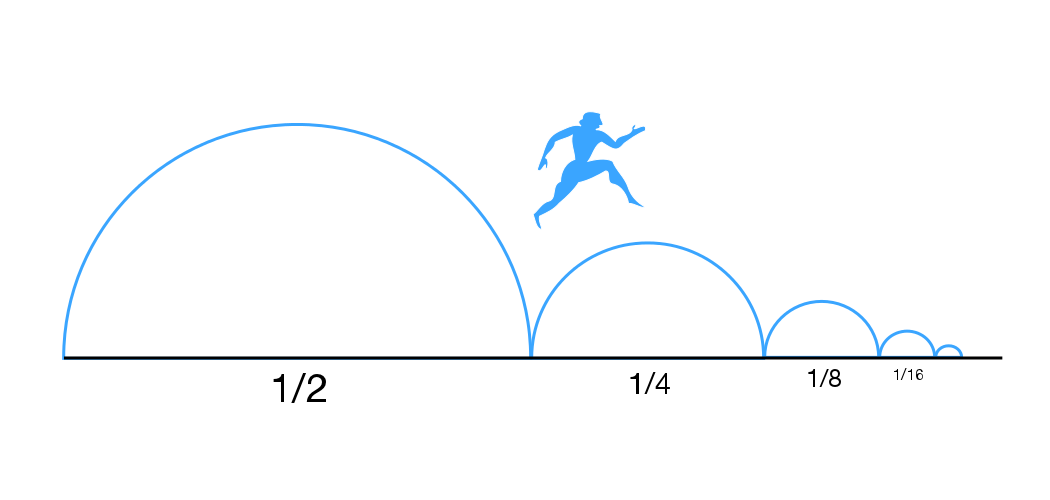We do a lot of proofs as part of this project, many of them using induction. In computer science, we’re usually taught that induction can prove facts about natural numbers, lists, trees, and other discrete structures. But in VeriDrone, we deal with the physical world, which means we work with real numbers. Can we use induction to prove facts about the real numbers? It turns out we can, though the induction rule is more subtle than you might expect.

### “Normal” Induction

Let’s say you want to prove a property $P$ of natural numbers. Induction says that you can prove $P$ holds on all natural numbers if you can prove

• $P$ holds on 0 and
• For any $n$, if $P$ holds on $n$, then $P$ holds on $n+1$.

Intuitively, this process resembles dominoes. We prove a fact for (knock over) the first element, and then use the inductive step to repeatedly prove the fact for (knock over) the next element.Now suppose we want to prove some other property $Q$ using induction, but $Q$ is a property of real numbers. Attempting to apply the same induction principle, we can prove that $Q$ holds on all non-negative real numbers if we can prove

• $Q$ holds on 0 and
• For any $x \geq 0$, if $Q$ holds on $x$, then $Q$ holds on ???

It’s not really clear how to complete the inductive step. What is the “next” real number after $x$? The real numbers are dense, which says that for any two real numbers that aren’t the same, there’s another real number between them. This means that there isn’t really a notion of a next element in the reals, as there is for natural numbers, lists, trees, and all the other structures we normally perform induction on. Real numbers are not like dominoes.

### Making it real

While there isn’t a next real number, we could consider the “next” element to be a non-empty interval of real numbers. Following this reasoning, the inductive step for real numbers would require one to prove

• For any $x \geq 0$, if $Q$ holds on $x$, then $Q$ holds on all $y \in [x,z)$ for some $z > x$

Unfortunately, this isn’t quite sufficient. The problem is that we can continue to take positive steps forward without ever covering all the real numbers. That is, we might only prove $Q$ for all real numbers less than some bound:There are a couple ways to resolve this issue. One is to ensure that each step is always at least as large as some positive constant. However, we’ll take an alternate approach. In this approach, we’ll make sure that if we prove $Q$ for all numbers less than some bound (e.g. 1 in the above image), then we prove it for that bound as well. Formally, this means we need a second inductive step:

• For any $x \geq 0$, if $Q$ holds on all $y \in [0,x)$, then $Q$ holds on $x$

To summarize, the full induction theorem states that we can prove that our friend $Q$ holds on all non-negative real numbers if we can prove:

1. $Q$ holds on 0
2. For any $x \geq 0$, if $Q$ holds on all $y \in [0,x]$, then $Q$ holds on all $y \in [x,z)$ for some $z > x$
3. For any $x \geq 0$, if $Q$ holds on all $y \in [0,x)$, then $Q$ holds on $x$

Note that we’ve weakened the first inductive step by assuming that $Q$ holds on all $y \in [0,x]$. This is possible for discrete induction as well.

The proof of real induction proceeds by contradiction. We construct the set of all elements not satisfying $Q$ and rely on the fact that such a set has a greatest lower bound. If the greatest lower bound satisfies $Q$ then this contradicts the first inductive step, and if it does not satisfy $Q$, then this contradicts the second inductive step.

The following is a more formal proof, following the proof style from this paper.

• Let $A=\{x \in \mathbb{R} ~:~ 0 \leq x \wedge \neg Q(x) \}$.
• Assume: $\exists~ x \in A$
• Prove: $False$
• ⟨1⟩1. $\exists~ i : \mathbb{R}$ such that $i$ is the greatest lower bound of $A$.
• Proof: All non-empty lower bounded sets of reals have a greatest lower bound.
• ⟨1⟩2. Case: $i\not\in A$.
• ⟨2⟩1. $\forall x \in [0, i],\ Q(x)$
• Proof: By the definition of greatest lower bound.
• ⟨2⟩2. $\exists~ z > i,\ \forall y \in [i, z),\ Q(y)$
• Proof: By ⟨2⟩1 and II.
• ⟨2⟩3. $z$ is a lower bound of $A$.
• Proof: By ⟨2⟩1 and ⟨2⟩2.
• ⟨2⟩4. QED
• Proof: By ⟨2⟩3 and the definition of greatest lower bound.
• ⟨1⟩3. Case: $i\in A$.
• ⟨2⟩1. $\forall ~ 0 \leq x < i,\ Q(x)$.
• Proof: Since $i$ is a lower bound of $A$
• ⟨2⟩2. $Q(i)$
• Proof: By III.
• ⟨2⟩3. QED
• Proof: By ⟨2⟩2 and ⟨1⟩3.
• ⟨1⟩4. QED
• Proof: By ⟨1⟩2 and ⟨1⟩3.

Of course, I didn’t come up with any of this myself. I took the theorem and proof from this paper, which mentions a number of other papers presenting some version of the theorem.

My esteemed colleague Gregory would also like me to mention that this result leverages the topology of the real numbers, though I’m not sure if anyone really knows what that means.

### An Application

The above paper gives many useful applications of real induction. Here, I’m going to illustrate how it works on a very simple example, inspired by the following simple application of discrete induction. Consider an infinite sequence of numbers $x_n$ where $x_0 \geq 0$ and $% $. Suppose we want to prove that $\forall n, x_n \geq 0$. We can do so by induction over the index into the sequence, which is a natural number.

Now let’s take a look at a continuous version of this property. Suppose we have some function $f : \mathbb{R} \rightarrow \mathbb{R}$ such that $0 \leq f(0)$. Moreover, suppose we know that $\forall t \geq 0, f'(t) > 0$. We would like to prove that $\forall t \geq 0, f(t) \geq 0$. As in the discrete case, we do so using induction, but this time using our induction principle for the reals. The base case (I) is trivial, so we need to prove two inductive steps. First,

• For any $x \geq 0$, if $f(y) \geq 0$ holds on all $y \in [0,x]$, then $f(y) \geq 0$ holds on all $y \in [x,z)$ for some $z > x$

This follows from the limit definition of a derivative. Informally, there is some neighborhood around $x$ such that for any point $y > x$ in that neighborhood, the slope of the line from $(x, f(x))$ to $(y, f(y))$ is between $0$ and $2*f'(x)$. In other words, the slope is nonnegative. More precisely, we instantiate $\epsilon$ in the limit definition with $f'(x)$ so that for any line, $|Slope - f'(x)| \leq f'(x)$. This implies that $f(y) \geq f(x)$, and $f(x)\geq 0$ by the induction hypothesis. The size of the neighborhood serves as $z-x$ in the above property. The following image depicts this:The second inductive step is

• For any $x \geq 0$, if $f(y) \geq 0$ holds on all $y \in [0,x)$, then $f(x) \geq 0$

This property follows from continuity of $f$. Suppose $% $. Then by continuity, there is some other point $% $ such that $% $, which is a contradiction.Of course, if we actually wanted to prove this property formally, we probably wouldn’t rely on such low-level definitions and would probably instead rely on general theorems about derivatives. Nevertheless, I hope this example helps to provide some intuition for real induction and a nice comparison with discrete induction.